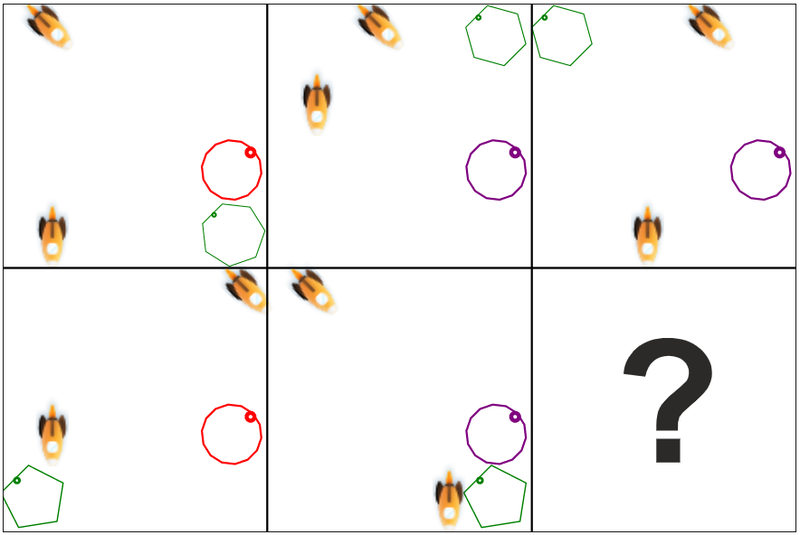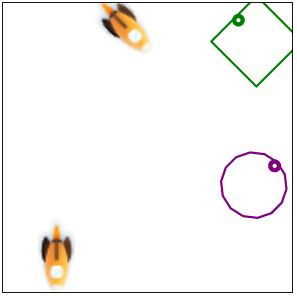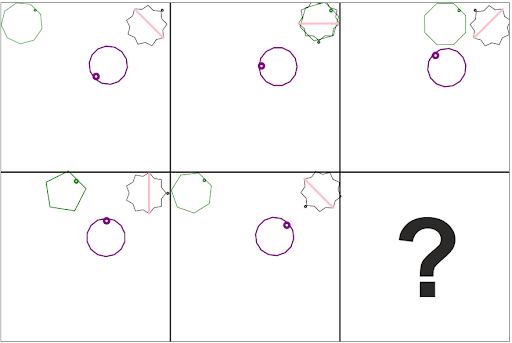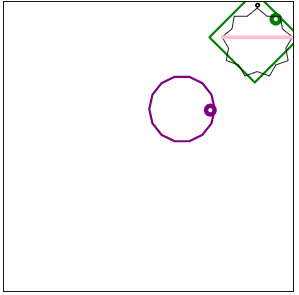# Figure Sequences ExplanationFigure Sequences

The category Figure Sequences tests how well you can recognize patterns and relationships in figures, this requires an abstract way of thinking. We have kept the explanation of this subject relatively short, because in this category it is especially important to practice. Only by practicing a lot, you will recognize the patterns that occur a lot and see how you can quickly solve the problems.

Below we will work out two examples to show you the best way to solve this type of problem. In addition, we offer many free exercise assignments to be able to enter your assessment with confidence.

Example 1

For example, you can see the five figures below. There is always a change pattern for each figure and you have to find out what changes need to be applied to get the correct answer. The series runs from top left to bottom right.Write down for yourself what patterns you can recognize. If you work systematically, you will make fewer mistakes and get to the correct answer faster.

• You can see that the circle changes from red to purple, then stays purple once and then turns red again. In the figure with the question mark, there must therefore be a purple circle in the same place as in the other figures.
• The rocket in the top left of figure 1 moves one position to the right every next figure. The position in the figure with the question mark must therefore be the same as in the second figure, because the rocket in figure 5 is in the same positions as in the initial figure.
• The green heptagon shifts an angle counterclockwise from each figure to the next. The position in the figure with the question mark must therefore be the same as in the second figure. In addition, an angle of the heptagon disappears every other time. In the figure with the question mark, there must therefore be a figure with 4 corners.
• The rocket in the bottom left of the first figure, first moves clockwise and then slightly further counterclockwise. This keeps repeating itself. In the figure with the question mark, the rocket must therefore be at the bottom left again.

The figure that has to replace the question mark will look like this:It often works best to not to look too much at the answers before you write down what patterns you can find. It is better to only start looking at the answers earlier if you are really under time pressure or you cannot find all the patterns without looking at the different possible answers.

Example 2It is often smart to start with the clearest patterns. In this case, for example, you can almost immediately see that the purple polygon rotates 45 degrees clockwise every step. In the final figure, the little dot should therefore be on the right side of the figure. For the rest, you can see the following changes:

• The figure that is in the top left of the first figure, always moves to the left in the horizontal plane. In addition, the number of angles changes. You have to look closely to see what changes between figures 1 and 2. In figure 1 the figure has 9 angles, in the second figure 6 angles, then 8 angles, then 5 angles, then 7 angles. You can see a pattern emerge in which three corners disappear first and then two more are added. So the figure with the question mark should have a green square in the top right corner.
• The star with the red stripe always remains in the same position. The figure rotates 135 degrees clockwise every step. In the final figure, the star must therefore be upright with the small dot at the top of the figure.

So the figure to replace the question mark will look like this:## Figure Sequences practice sets